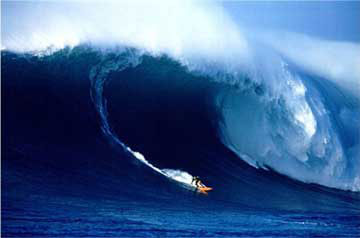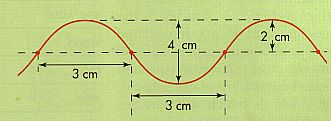# Properties of Waves## Worksheet A

What are the features of waves? How do we categorise wave types? How do waves move?

In this activity you will use the internet to understand the features and characteristics of waves. You will learn the terminology we use to describe the features of waves and how waves move.

Use the Internet

1. Work individually for this task
2. Visit the following website to find your information:

The Physics Classroom

Descriptive Questions:

1. Describe the difference between a longitudinal and transverse type wave. Give one example of each type of wave.

2. Light is an electromagnetic wave that is considered to be transverse in nature. What feature of this wave type suggests it is a transverse wave?

3. Describe each of the following waves as propagating in one, two or three dimensions:

(a) the light emitted from the Sun

(b) sound from a bell

(c) a sound wave travelling along a string telephone made from a tight string and two tin cans

(d) a water wave produced by dropping a rock into the centre of a lake

4. Explain what is the frequency of a wave. Is frequency the same as the period of a wave? If not, then describe how they are related.

5. Describe what is the speed of a wave. Explain how the speed of a wave is related to the frequency and wavelength of a wave.

Problem solving Questions:

1. Look at the picture shown below of a transverse wave:This transverse wave is travelling at a speed of 14 metres per second. For this transverse wave calculate the following values:

(a) The wavelength

(b) The amplitude

(c) The frequency

(d) The period

2. If eight water waves pass an ocean buoy each minute, and successive wave crests are 20 m apart, find the wave velocity.

3. A Sydney radio station (MIX) broadcasts on 106.5 MHz. Calculate the length of the transmitter antenna in order for it to be exactly one half the wavelength of this transmission signal.

4. If the blue light reaching earth is from a blue star with a wavelength of 410 nm, calculate the frequency of the light.

5. Fifty compressions of air pass a point every second. If the speed of sound is 340 m/s, find the frequency and wavelength of this wave.

6. The wavelength of certain red light in air is 624 nm.

(a) Calculate its frequency

(b) If this light enters water its speed is cut down by 25% but its frequency is unaltered. Calculate the value of its wavelength in water.

Congratulations you have now completed this activity worksheet. Check all your answers carefully, enter your name and your teacher's name in the text boxes below and finally print out this webpage to hand in to your teacher.